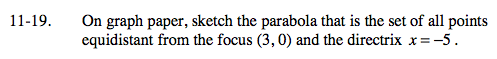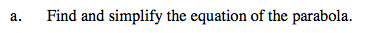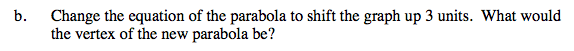### Home > A2C > Chapter Ch11 > Lesson 11.1.2 > Problem11-19

11-19.
1. On graph paper, sketch the parabola that is the set of all points equidistant from the focus (3, 0) and the directrix x = −5. Homework Help ✎

1. Find and simplify the equation of the parabola.

2. Change the equation of the parabola to shift the graph up 3 units. What would the vertex of the new parabola be?Find the expressions for the distance from the directrix to the point (x, y) and the distance from the focus to the same point, then set both of them equal to each other.

$\sqrt{(x - 3)^2 + y^2} = x + 5$

Now simplify.Subtract 3 from y before squaring to shift the graph up 3 units.